2021年3月26日

# # 排序 - 计数排序(Counting Sort)详解

## # 计数排序基础版

### # 基础版算法步骤

• 第1步：找出原数组中元素值最大的，记为max。
• 第2步：创建一个新数组count，其长度是max加1，其元素默认值都为0。
• 第3步：遍历原数组中的元素，以原数组中的元素作为count数组的索引，以原数组中的元素出现次数作为count数组的元素值。
• 第4步：创建结果数组result，起始索引index。
• 第5步：遍历count数组，找出其中元素值大于0的元素，将其对应的索引作为元素值填充到result数组中去，每处理一次，count中的该元素值减1，直到该元素值不大于0，依次处理count中剩下的元素。
• 第6步：返回结果数组result。

### # 基础版代码实现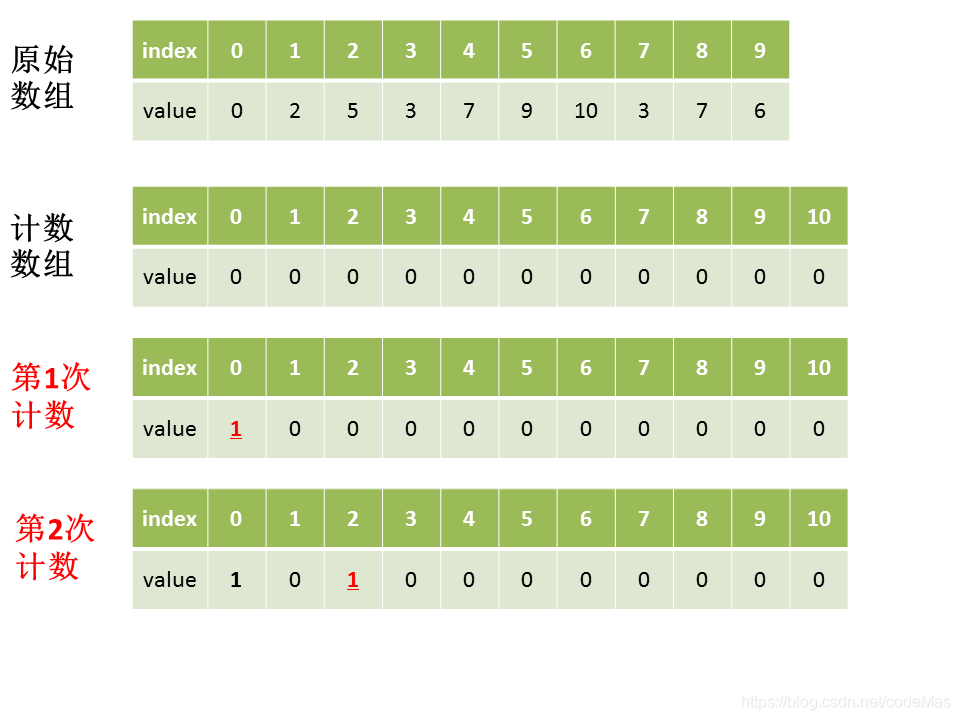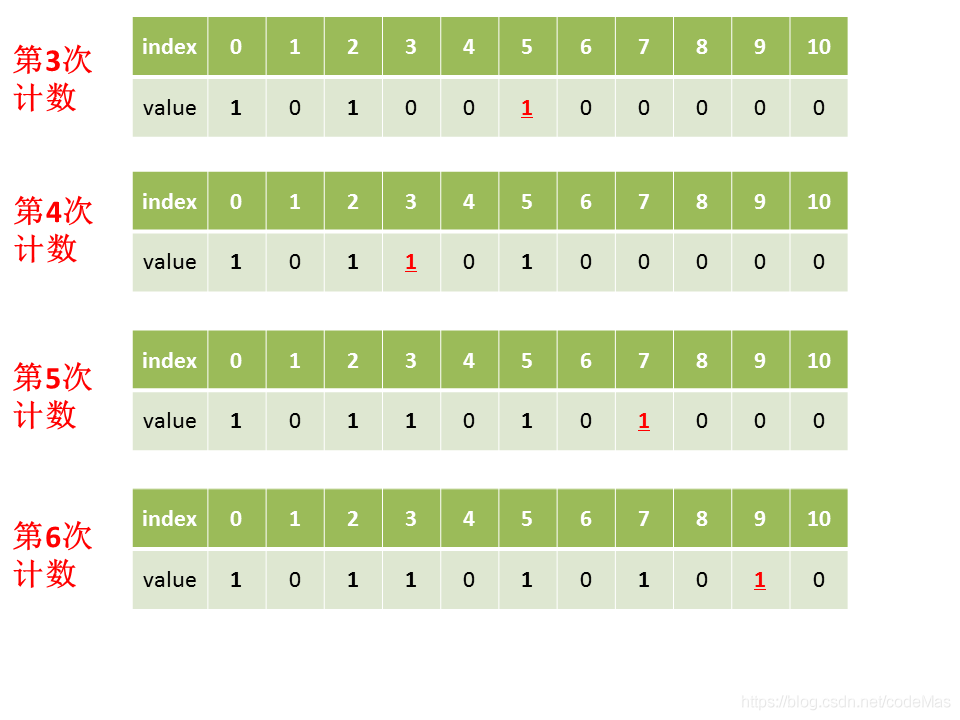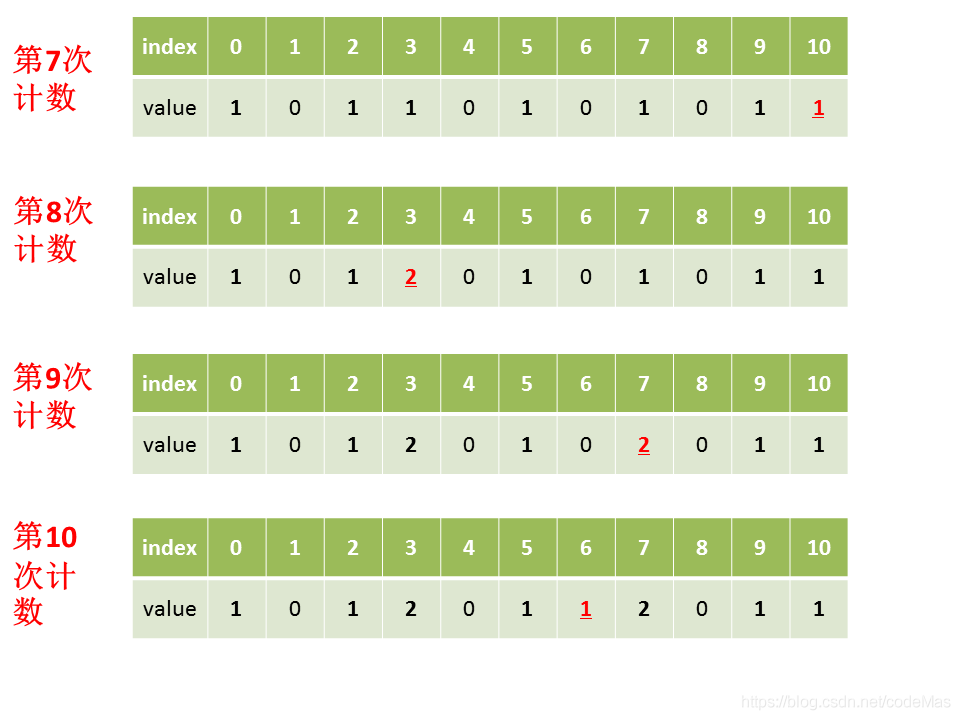``````public int[] countSort(int[] A) {
// 找出数组A中的最大值
int max = Integer.MIN_VALUE;
for (int num : A) {
max = Math.max(max, num);
}
// 初始化计数数组count
int[] count = new int[max+1];
// 对计数数组各元素赋值
for (int num : A) {
count[num]++;
}
// 创建结果数组
int[] result = new int[A.length];
// 创建结果数组的起始索引
int index = 0;
// 遍历计数数组，将计数数组的索引填充到结果数组中
for (int i=0; i<count.length; i++) {
while (count[i]>0) {
result[index++] = i;
count[i]--;
}
}
// 返回结果数组
return result;
}
``````

## # 计数排序优化版

``````public int[] countSort2(int[] A) {
// 找出数组A中的最大值、最小值
int max = Integer.MIN_VALUE;
int min = Integer.MAX_VALUE;
for (int num : A) {
max = Math.max(max, num);
min = Math.min(min, num);
}
// 初始化计数数组count
// 长度为最大值减最小值加1
int[] count = new int[max-min+1];
// 对计数数组各元素赋值
for (int num : A) {
// A中的元素要减去最小值，再作为新索引
count[num-min]++;
}
// 创建结果数组
int[] result = new int[A.length];
// 创建结果数组的起始索引
int index = 0;
// 遍历计数数组，将计数数组的索引填充到结果数组中
for (int i=0; i<count.length; i++) {
while (count[i]>0) {
// 再将减去的最小值补上
result[index++] = i+min;
count[i]--;
}
}
// 返回结果数组
return result;
}
``````

## # 计数排序进阶版

### # 进阶版算法步骤

• 第1步：找出数组中的最大值max、最小值min。
• 第2步：创建一个新数组count，其长度是max-min加1，其元素默认值都为0。
• 第3步：遍历原数组中的元素，以原数组中的元素作为count数组的索引，以原数组中的元素出现次数作为count数组的元素值。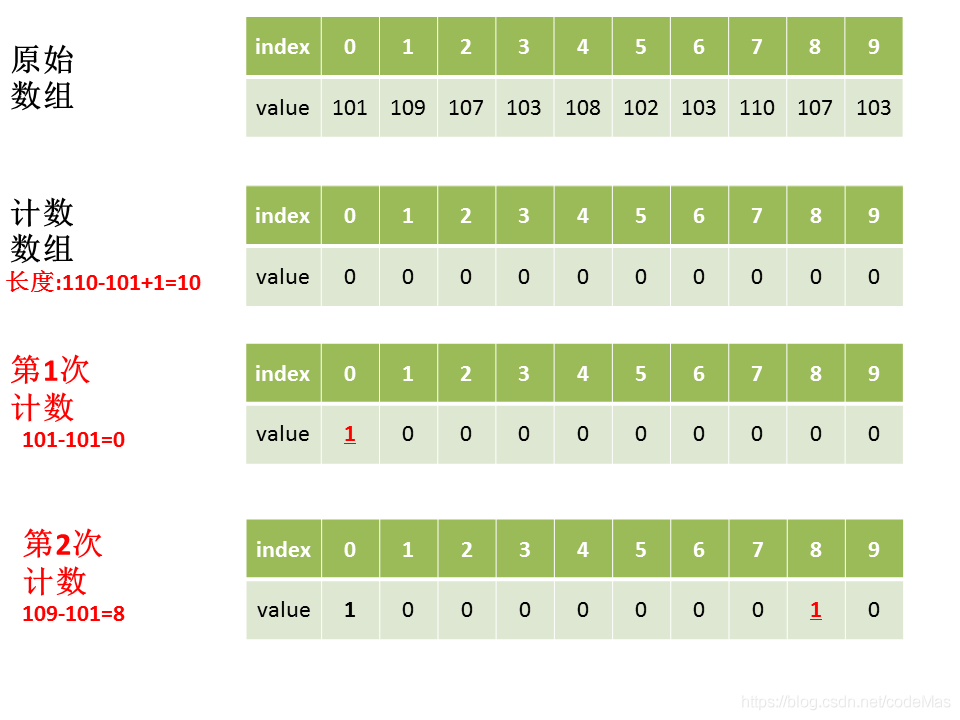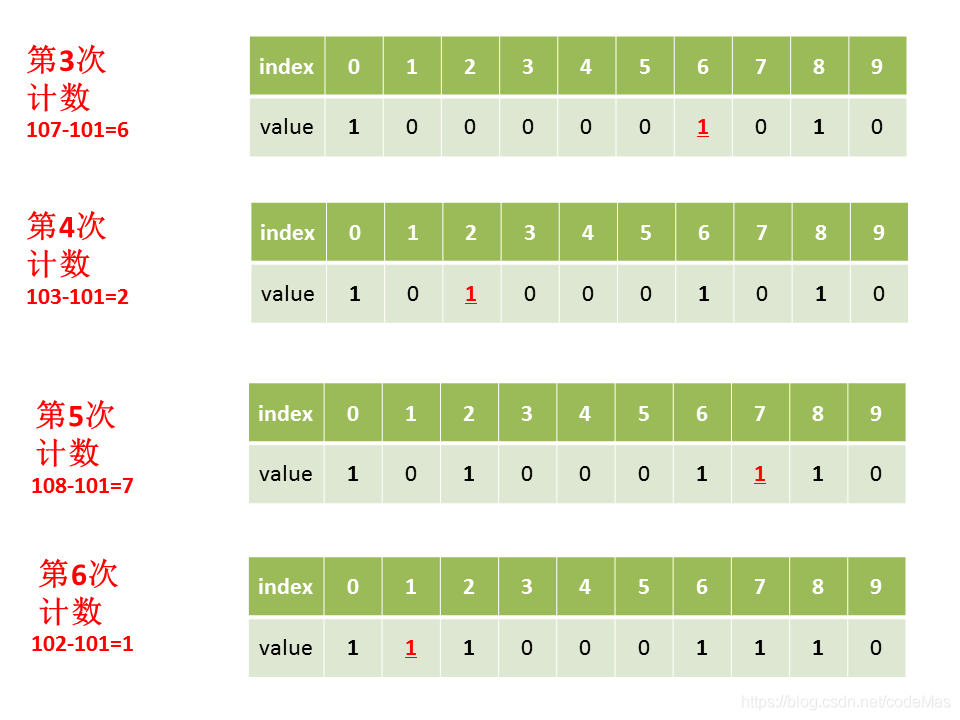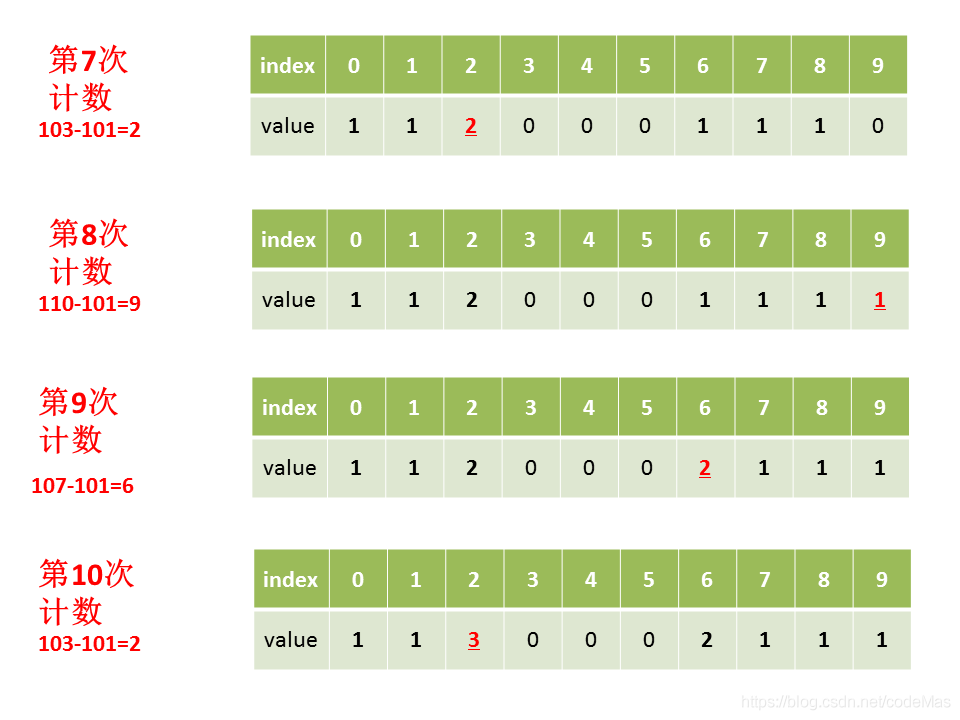• 第4步：对count数组变形，新元素的值是前面元素累加之和的值，即`count[i+1] = count[i+1] + count[i];`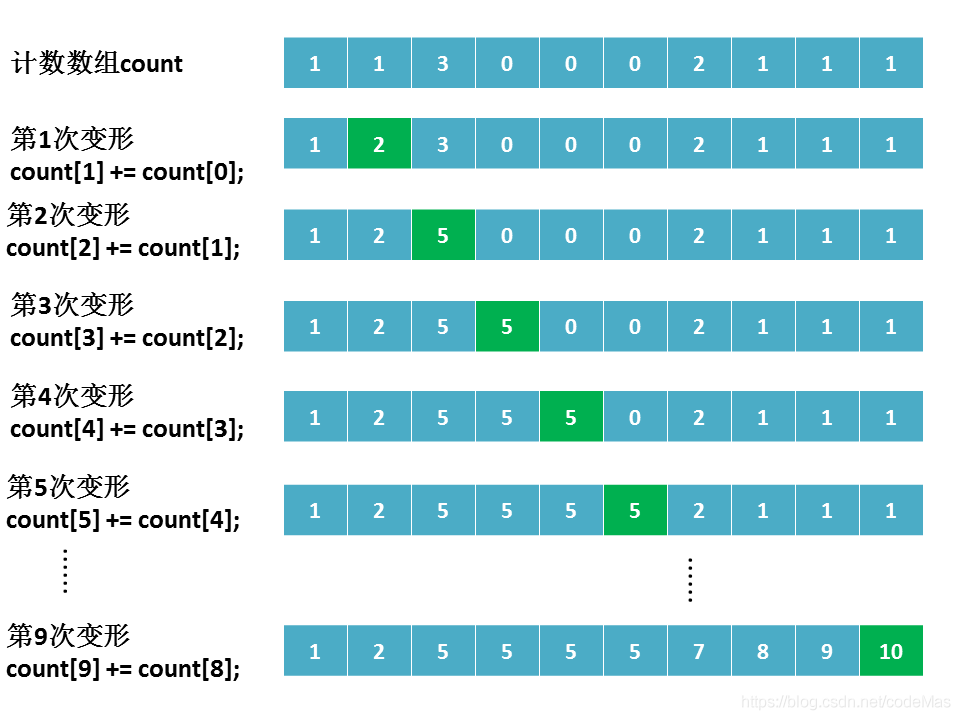• 第5五步：创建结果数组result，长度和原始数组一样。
• 第6步：遍历原始数组中的元素，当前元素`A[j]`减去最小值min，作为索引，在计数数组中找到对应的元素值`count[A[j]-min]`，再将`count[A[j]-min]`的值减去1，就是`A[j]`在结果数组result中的位置，做完上述这些操作，`count[A[j]-min]`自减1。

### # 进阶版代码实现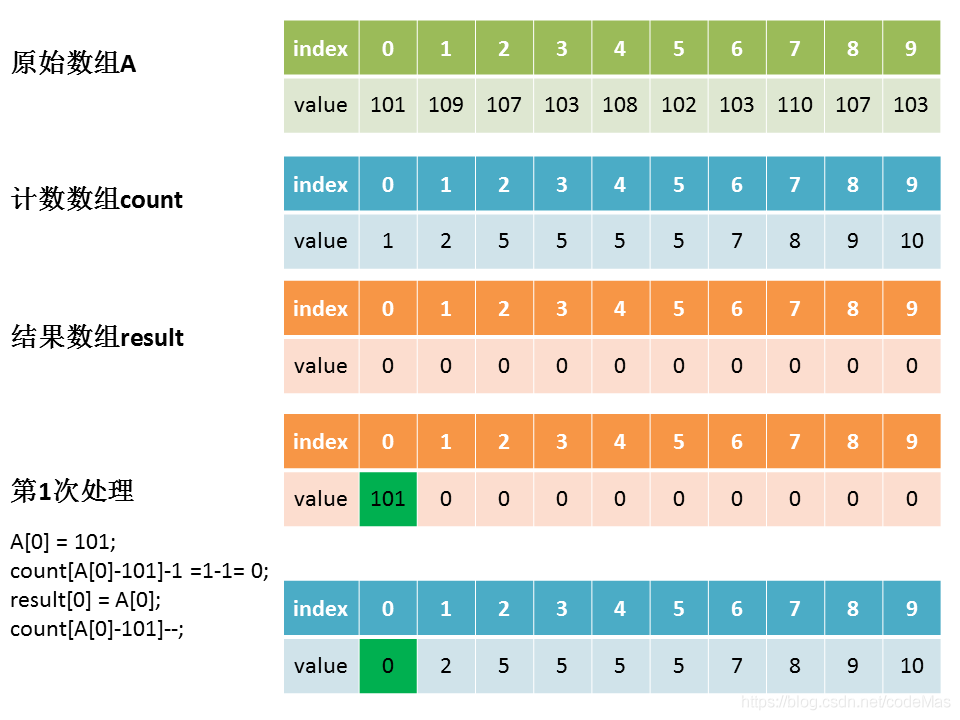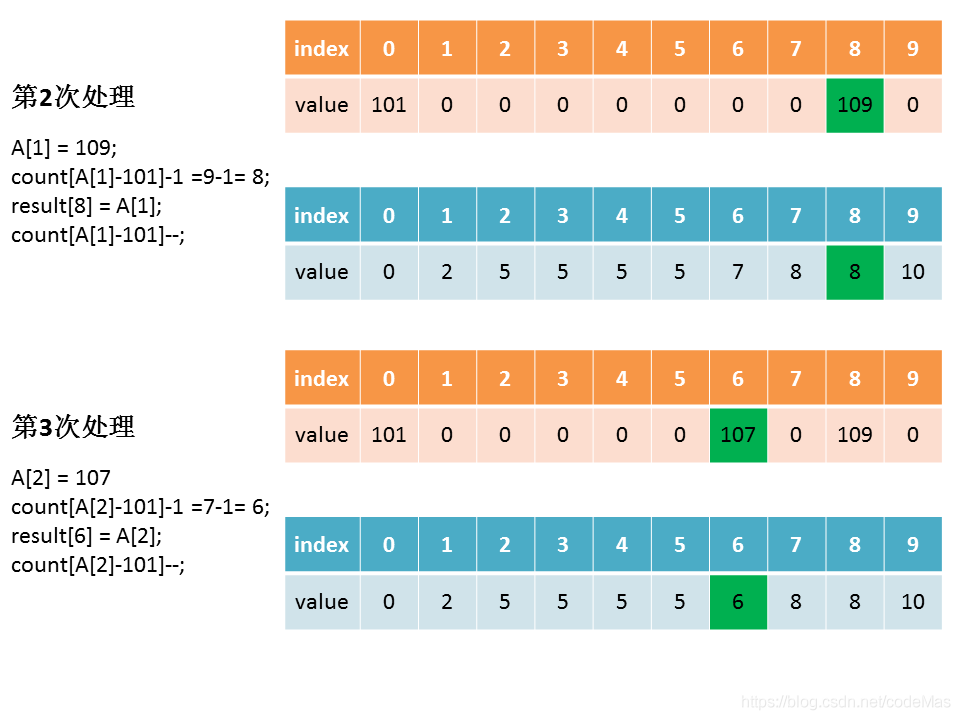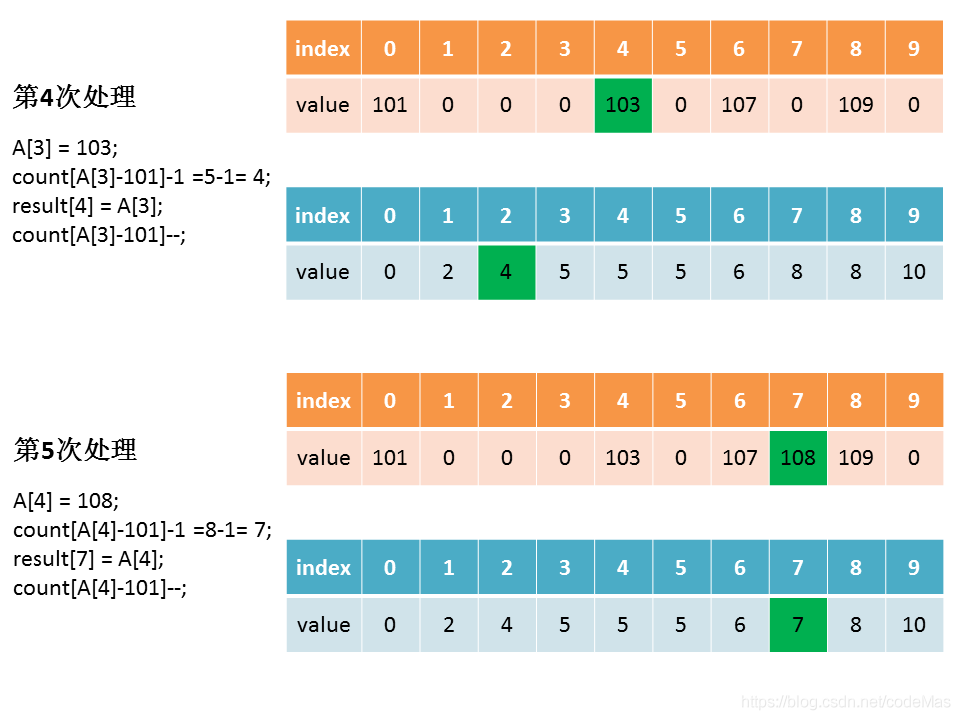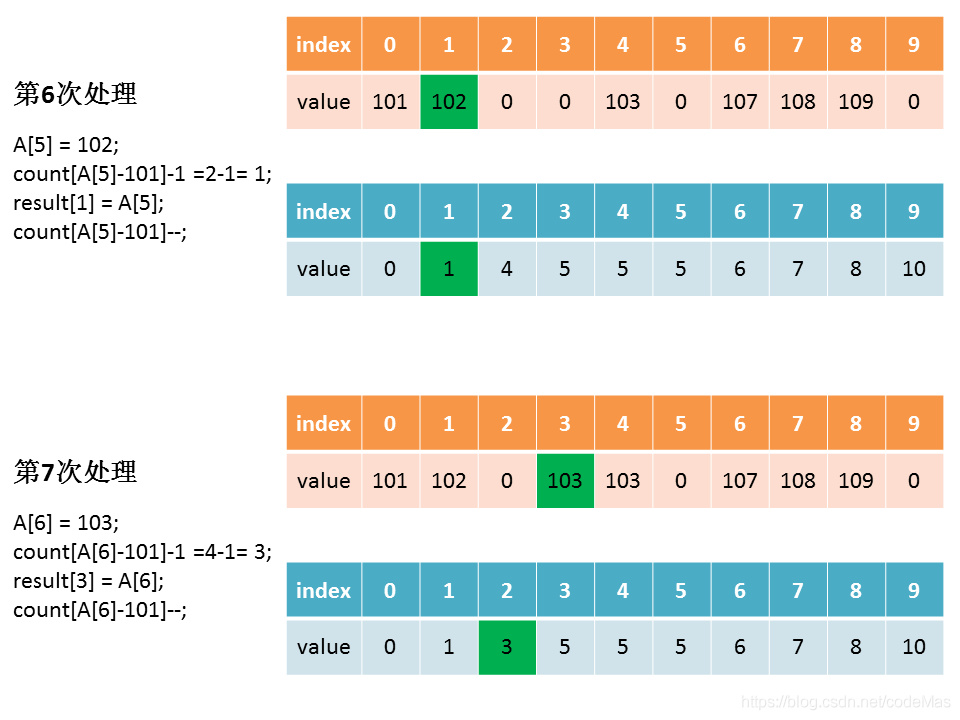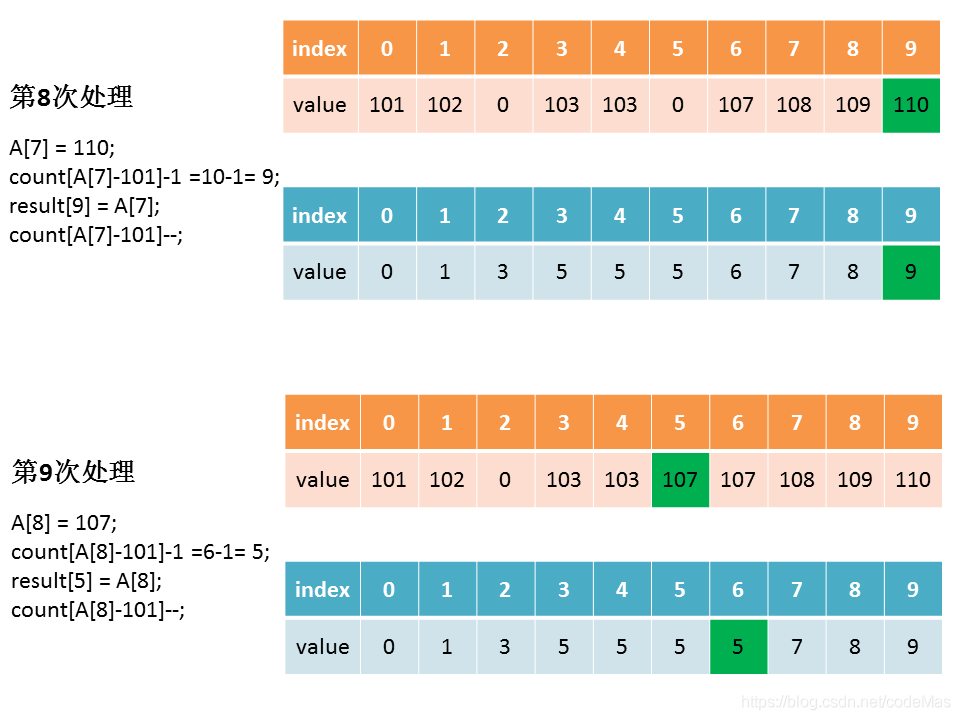``````public int[] countSort3(int[] A) {
// 找出数组A中的最大值、最小值
int max = Integer.MIN_VALUE;
int min = Integer.MAX_VALUE;
for (int num : A) {
max = Math.max(max, num);
min = Math.min(min, num);
}
// 初始化计数数组count
// 长度为最大值减最小值加1
int[] count = new int[max-min+1];
// 对计数数组各元素赋值
for (int num : A) {
// A中的元素要减去最小值，再作为新索引
count[num-min]++;
}
// 计数数组变形，新元素的值是前面元素累加之和的值
for (int i=1; i<count.length; i++) {
count[i] += count[i-1];
}
// 创建结果数组
int[] result = new int[A.length];
// 遍历A中的元素，填充到结果数组中去
for (int j=0; j<A.length; j++) {
result[count[A[j]-min]-1] = A[j];
count[A[j]-min]--;
}
return result;
}
``````

### # 进阶版延伸之一

``````public int[] countSort4(int[] A) {
// 找出数组A中的最大值、最小值
int max = Integer.MIN_VALUE;
int min = Integer.MAX_VALUE;
for (int num : A) {
max = Math.max(max, num);
min = Math.min(min, num);
}
// 初始化计数数组count
// 长度为最大值减最小值加1
int[] count = new int[max-min+1];
// 对计数数组各元素赋值
for (int num : A) {
// A中的元素要减去最小值，再作为新索引
count[num-min]++;
}
// 计数数组变形，新元素的值是前面元素累加之和的值
for (int i=1; i<count.length; i++) {
count[i] += count[i-1];
}
// 创建结果数组
int[] result = new int[A.length];
// 遍历A中的元素，填充到结果数组中去，从后往前遍历
for (int j=A.length-1; j>=0; j--) {
result[count[A[j]-min]-1] = A[j];
count[A[j]-min]--;
}
return result;
}
``````

### # 进阶版延伸之二

• 第1步：找出数组中的最大值max、最小值min。
• 第2步：创建一个新数组count，其长度是max-min加1再加1，其元素默认值都为0。
• 第3步：遍历原数组中的元素，以原数组中的元素作为count数组的索引，以原数组中的元素出现次数作为count数组的元素值。
• 第4步：对count数组变形，新元素的值是前面元素累加之和的值，即`count[i+1] = count[i+1] + count[i];`
• 第5步：创建结果数组result，长度和原始数组一样。
• 第6步：从前往后遍历原始数组中的元素，当前元素`A[j]`减去最小值min，作为索引，在计数数组中找到对应的元素值`count[A[j]-min]`，就是`A[j]`在结果数组result中的位置，做完上述这些操作，`count[A[j]-min]`自增加1。

``````public int[] countSort5(int[] A) {
// 找出数组A中的最大值、最小值
int max = Integer.MIN_VALUE;
int min = Integer.MAX_VALUE;
for (int num : A) {
max = Math.max(max, num);
min = Math.min(min, num);
}
// 初始化计数数组count
// 长度为最大值减最小值加1，再加1
int[] count = new int[(max-min+1)+1];
// 对计数数组各元素赋值，count永远为0
for (int num : A) {
// A中的元素要减去最小值再加上1，再作为新索引
count[num-min+1]++;
}
// 计数数组变形，新元素的值是前面元素累加之和的值
for (int i=1; i<count.length; i++) {
count[i] += count[i-1];
}
// 创建结果数组
int[] result = new int[A.length];
// 遍历A中的元素，填充到结果数组中去，从前往后遍历
for (int j=0; j<A.length; j++) {
// 如果后面遇到相同的元素，在前面元素的基础上往后排
// 如此就保证了原始数组中相同元素的原始排序
result[count[A[j]-min]] = A[j];
count[A[j]-min]++;
}
return result;
}
``````

## # 计数排序总结

• 一是需要排序的元素必须是整数；
• 二是排序元素的取值要在一定范围内，并且比较集中。

## # 引用资料

• https://www.cnblogs.com/xiaochuan94/p/11198610.html